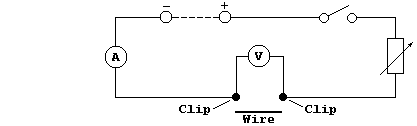```EXPERIMENTAL: AN INVESTIGATION INTO THE DEPENDENCE OF ELECTRICAL
RESISTANCE ON THE DIAMETER AND ON THE LENGTH OF NICHROME WIRE
Introduction
The resistance of a conductor is well known to be dependent upon the
material it is made from, its length, diameter, and temperature: but,
quantitative relationships between the variables need to be determined
by controlled experiments ...```
 ```In this investigation, using the so-called voltmeter-ammeter method, you are required to examine the dependence of resistance (R) on the diameter (D) and on the length (L) of Nichrome wire - in particular, to determine the validity of the following relationships: R = k ◊ D + c; R = k ◊ D-Ļ + c; R = k ◊ L + c; R = k ◊ L-Ļ + c.```
```Method
1.  Construct the circuit shown below, with the d.c. output set to a
constant value (about 4.5 V ?).``````2.  Measure 0.270 m of Ni-Cr resistance wire of one chosen diameter; i
this value is not known, the wire should be measured with a micrometer.
Place the inside of each crocodile clip 0.010 m away from each end of
the wire, so that the effective length of wire is 0.250 m.
3.  Close the switch and immediately record the readings of both the
ammeter and the voltmeter; then open the switch.
4.  Using an effective length of 0.250 m, repeat steps 2 and 3 for each
diameter wire (within the range 0.28 - 0.56 mm) and, after examining
the raw data, for appropriate duplicates.
5.  Finally, for wire of one chosen diameter, modify steps 2 to 4 so as
to obtain similar data for differing lengths of wire (within the range
of 0.100 - 0.400 m).
Tables of Results and Calculations```
 ```Constants (for independent variables D and D-Ļ): ___________________ ____________________________________________________________________ ____________________________________________________________________ ____________________________________________________________________``` ``` Diameter (D) / mm``` ``` Diameter-Ļ (D-Ļ) / mm-Ļ``` ``` Voltage (V) / V``` ``` Current (I) / A``` ``` Resistance (R) / W``` ``` Power (P) / W``` ` ` ` ` ` ` ` ` ` ` ` ` ` ` ` ` ` ` ` ` ` ` ` ` ` ` ` ` ` ` ` ` ` ` ` ` ` ` ` ` ` ` ` ` ` ` ` ` ` ` ` ` ` ` ` ` ` ` ` ` ` ` ` ` ` ` ` ` ` ` ` `
`    [Resistance = Voltage ų Current      Power = Voltage ◊ Current]`
 ```Constants (for independent variables L and L-Ļ): ___________________ ____________________________________________________________________ ____________________________________________________________________ ____________________________________________________________________``` ``` Length (L) / m``` ``` Length-Ļ (L-Ļ) / m-Ļ``` ``` Voltage (V) / V``` ``` Current (I) / A``` ``` Resistance (R) / W``` ``` Power (P) / W``` ` ` ` ` ` ` ` ` ` ` ` ` ` ` ` ` ` ` ` ` ` ` ` ` ` ` ` ` ` ` ` ` ` ` ` ` ` ` ` ` ` ` ` ` ` ` ` ` ` ` ` ` ` ` ` ` ` ` ` ` ` ` ` ` ` ` ` ` ` ` ` `
```Notes (and Extension Work)
1.  Complete this investigation, as follows.  First, construct a graph
for each independent variable; you will need to truncate both axes for
each graph.  Second, write a critical evaluation.  Third, construct a
precisely worded conclusion for each of the investigated relationships.
And fourth, suggest one or more interpretations of the results.
2.  The wires used in the National Grid system carry very high voltages
and fairly high currents over long distances, so they must be thick in
order to minimize losses of 'low-grade' heat energy; therefore, cheap,
low-density aluminium is used rather than expensive, high-density
copper (although this metal has a lower resistance, and so lower losses
in conducting electrical energy).  Consider investigating some aspects
of 'resistance heating', by examining the dependence of power (P) on
various independent variables (... using a graph/statistics program?).
Dr. R. Peters                  Next                      Contents' List```